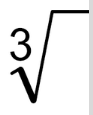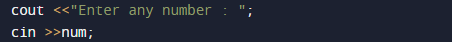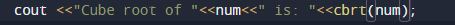C++ Programs to Find Cube Root of Number

In this tutorial you will learn about the C++ Programs to Find Cube Root of Number and its application with practical example.

In this tutorial, we will learn to create a C++ program that will find Cube Root of given number using C++ programming.

Prerequisites

Before starting with this tutorial we assume that you are best aware of the following C ++ programming topics:

• Operators.
• Basic input/output.
• In-built functions.
• mathematical functions.

What Is cube root?

Cube root , is a factor that we multiply by itself three times to get that number (2*2*2=8).A number which produces  a number when cubed.C++ Programs to Find Cube Root of Number.

Firstly we declare required header file and variable and also initiates required values in variable. Here in this program we are going find cube root of a given number first of all we take a value from user and pass this value to in-built function to find cube root of a number.

OutputFunction cbrt() takes only single  parameter(num) and returns the cube root.which we hold in cube_root variable.

In the above program, we have first declared and initialized a set variables required in the program.

• num = it will hold  given number.with the help of cbrt() in-built function we can find the cube of given number of user.

Step by step explanation of this program.

1:First we declare  variable num.
2 :Then  take a value from user and assign to num.3 : Pass no variable to cube_root function (cbrt(num)).
4 : This function returns value to cube root .
5 : Print the value of cube_root.Explanation of Cube root, cube root of a number is a value ,when multiplied by itself 3 times . For example, the cube root of 27, denoted as 3√27, is 3, because when we multiply 3 by itself thrice times we get 3 x 3 x 3 = 27 = 33.

In this tutorial we have learn about the C++ Programs to Find Cube Root of Number and its application with practical example. I hope you will like this tutorial.